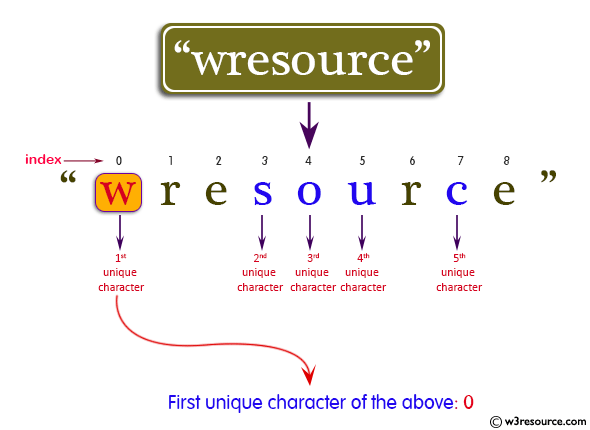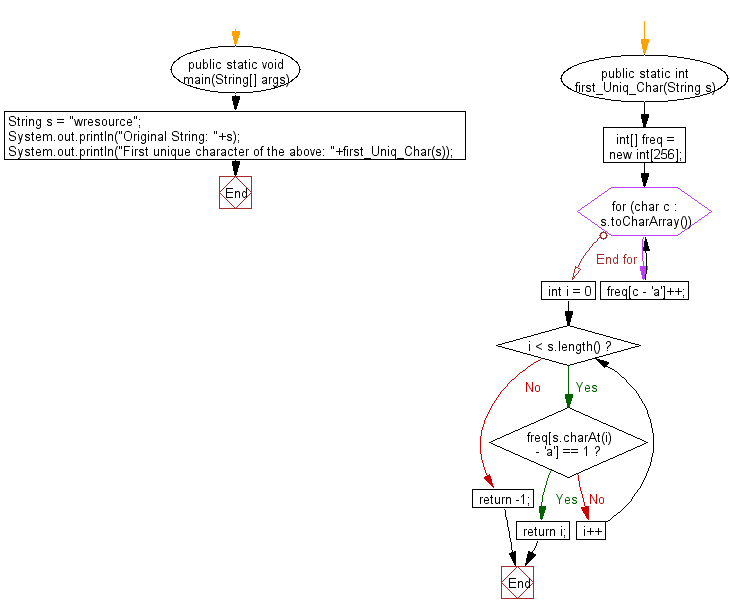﻿ Java: Find index of the first unique character in a string# Java Exercises: Find the index of the first unique character in a given string

## Java Basic: Exercise-148 with Solution

Write a Java program to find the index of the first unique character in a given string, assume that there is at least one unique character in the string.

Pictorial Presentation:Sample Solution:

Java Code:

``````import java.util.*;
public  class  Solution {
public static void main(String[] args) {
String s = "wresource";
System.out.println("Original String: "+s);
System.out.println("First unique character of the above: "+first_Uniq_Char(s));
}

public static int first_Uniq_Char(String s) {
int[] freq = new int;
for (char c : s.toCharArray()) {
freq[c - 'a']++;
}
for (int i = 0; i < s.length(); i++) {
if (freq[s.charAt(i) - 'a'] == 1) return i;
}
return -1;
}
}
```
```

Sample Output:

```Original String: wresource
First unique character of the above: 0
```

Flowchart:Java Code Editor:

Company:

What is the difficulty level of this exercise?

Test your Programming skills with w3resource's quiz.

﻿

## Java: Tips of the Day

getEnumMap

Converts to enum to Map where key is the name and value is Enum itself.

```public static <E extends Enum<E>> Map<String, E> getEnumMap(final Class<E> enumClass) {
return Arrays.stream(enumClass.getEnumConstants())
.collect(Collectors.toMap(Enum::name, Function.identity()));
}
```

Ref: https://bit.ly/3xXcFZt